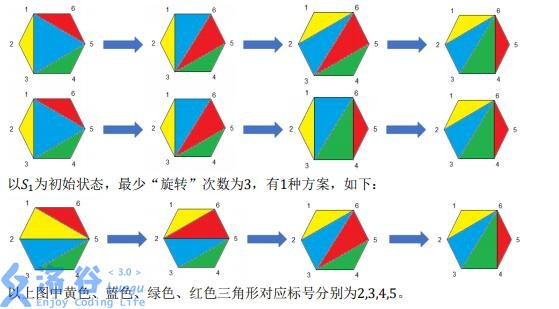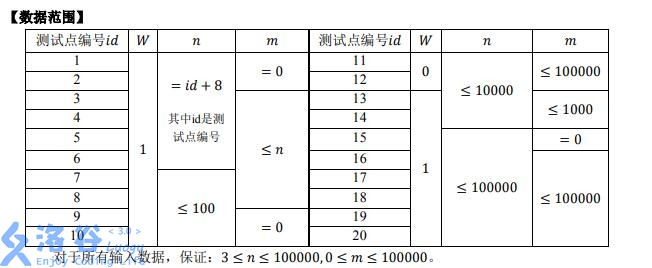# P5288 [HNOI2019]多边形

• 110通过
• 278提交
• 题目提供者 chen_zhe Aya
• 评测方式 云端评测
• 标签 各省省选 2019 湖南
• 难度 NOI/NOI+/CTSC
• 时空限制 1000ms / 512MB
• 提示：收藏到任务计划后，可在首页查看。

## 题目描述

小 R 与小 W 在玩游戏。

他们有一个边数为$n$的凸多边形，其顶点沿逆时针方向标号依次为$1,2,3,\cdots , n$。最开始凸多边形中有$n$条线段，即多边形的$n$条边。这里我们用一个有序数对$(a, b)$（其中$a < b$）来表示一条端点分别为顶点$a,b$的线段。

在游戏开始之前，小 W 会进行一些操作。每次操作时，他会选中多边形的两个互异顶点，给它们之间连一条线段，并且所连的线段不会与已存的线段重合、相交（只拥有一个公共端点不算作相交）。

他会不断重复这个过程，直到无法继续连线，这样得到了状态$s_0$。$s_0$包含的线段为凸多边形的边与小W 连上的线段，容易发现这些线段将多边形划分为一个个三角形区域。对于其中任意一个三角形，其三个顶点为$i,j,k(i < j < k)$，我们可以给这个三角形一个标号$j$，这样一来每个三角形都被标上了$2,3, \cdots , n - 1$中的一个，且没有标号相同的两个三角形。

小 W 定义了一种“旋转”操作：对于当前状态，选定$4$个顶点$a,b,c,d$，使其满足$1 \leq a < b < c <d \leq n$且它们两两之间共有$5$条线段——$(a,b), (b,c), (c,d), (a,d), (a,c)$，然后删去线段$(a,c)$，并连上线段$(b,d)$。那么用有序数对$(a,c)$即可唯一表示该次“旋转”。我们称这次旋转为$(a,c)$ “旋转”。显然每次进行完“旋转”操作后多边形中依然不存在相交的线段。

当小 W 将一个状态作为游戏初始状态展示给小 R 后，游戏开始。游戏过程中，小 R 每次可以对当前的状态进行“旋转”。在进行有限次“旋转”之后，小 R 一定会得到一个状态，此时无法继续进行“旋转”操作，游戏结束。那么将每一次“旋转”所对应的有序数对按操作顺序写下，得到的序列即为该轮游戏的操作方案。

为了加大难度，小 W 以$s_0$为基础，产生了$m$个新状态。其中第$i$个状态$s_i$为对$s_0$进行一次“旋转”操作后得到的状态。你需要帮助小 R 求出分别以$s_0,s_1\cdots s_n$作为游戏初始状态时，小 R 完成游戏所用的最少“旋转”次数，并根据小 W 的心情，有时还需求出“旋转”次数最少的不同操作方案数。由于方案数可能很大，输出时请对$1e9+7$取模。

## 输入输出格式

输入格式：

第一行一个整数$W$，表示小W的心情。若$W$为$0$则只需求出最少的“旋转”次数，若$W$为$1$则还需求出“旋转”次数最少时的不同操作方案数。

第二行一个正整数$n$，表示凸多边形的边数。

接下来$n-3$行，每行两个正整数$x,y$，表示小W在$s_0$中连的一条线段，端点分别为$x,y$。保证该线段不与已存的线段重合或相交。

接下来一行一个整数$m$，表示小W以$s_0$为基础产生的新状态个数。

接下来$m$行，每行两个整数。假设其中第$i$行为$a,b$，表示对$s_0$进行$(a,b)$“旋转”后得到$s_i$。

输出格式：

输出共$m+1$行。

若$W$为$0$则每一行输出一个整数，第$i(i = 1,2, \cdots , m, m + 1)$行输出的整数表示$S_{i-1}$作为初始局面的最少“旋转”次数。

若$W$为$1$则每一行输出两个整数，第$i(i = 1,2, \cdots , m, m + 1)$行输出的两个整数依次表示$S_{i-1}$作为初始局面的最少“旋转”次数、“旋转”次数最少的不同操作方案数对$1e9+7$取模的结果。

## 输入输出样例

输入样例#1： 复制
1
6
1 3
1 5
3 5
1
1 3
输出样例#1： 复制
3 2
3 1

输入样例#2： 复制
1
12
1 10
1 6
1 3
3 6
3 5
6 10
6 8
8 10
10 12
4
1 10
1 3
6 8
1 6
输出样例#2： 复制
8 210
7 210
8 70
8 105
8 140

## 说明提示
标程仅供做题后或实在无思路时参考。
请自觉、自律地使用该功能并请对自己的学习负责。
如果发现恶意抄袭标程，将按照I类违反进行处理。